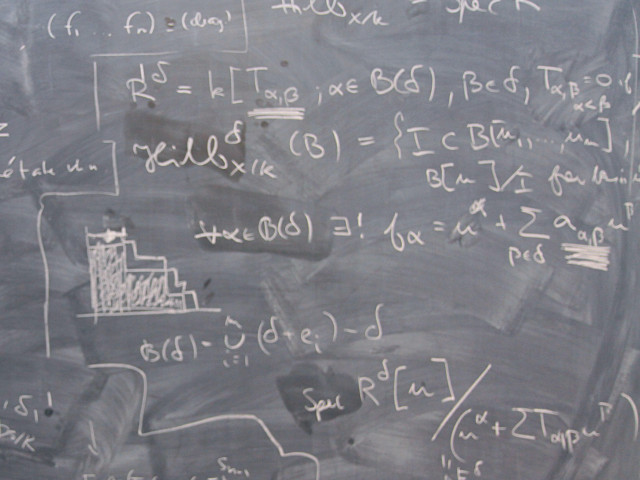# SF1629 Differential Equations and Transforms II 9.0 creditsA course of (mostly) ordinary differential equations and transform methods, including Fourier series.

Course offerings are missing for current or upcoming semesters.
Headings with content from the Course syllabus SF1629 (Autumn 2013–) are denoted with an asterisk ( )

## Content and learning outcomes

### Course contents

First order differential equations. Second order linear equations. The Laplace transform. Systems of differential equations. Qualitative methods for non-linear differential equations. Analysis at critical points. Long termbehaviour.  Stability. Existence- and uniqueness theorems.
Fourier series, orthogonal systems of functions. Sturm-Liouville problems.
The Fourier transform. Discrete transforms. Distributions. Partial differential equations. Separation of variables. Applications to ordinary and partial differential equations.

### Intended learning outcomes

After the course, the students should be able to

• solve first order ordinary differential equations (especially separable, linear and exact equations)
• solve second order linear differential equations using reduction of order and variation of parameters
• solve second order linear differential equations using power series
• solve differential and integral equations using Laplace transforms
• solve systems of first order linear differential equations, classify critical points of autonomous systems, determine the trajectories and phase portraits for autonomous systems and investigate the stability of critical points (especially by linearization)
• calculate Fourier series and their sums
• use summation kernels
• solve approximation problems using orthogonal projections in inner product spaces
• solve problems using systems of orthogonal polynomials
• solve partial differential equations using separation of variables
• solve the Dirichlet problem in the unit disc
• solve Sturm-Liouville problems
• calculate Fourier transforms, use Fourier transforms and convolutions in order to carry out computations (including applications to partial differential equations) and use the Z-transform
• carry out computations using distributions and their derivatives and Fourier Transforms

### Course disposition

No information inserted

## Literature and preparations

### Specific prerequisites

SF1602 + SF1603 Differential and integral calculus II, parts 1 and 2, as well as SF1604 Linear Algebra.

### Recommended prerequisites

No information inserted

### Equipment

No information inserted

### Literature

Boyce-Diprima:Elementary Differential Equations and Boundary Value Problems, 10:th ed.

Anders Vretblad: FOURIERANALYSIS and Its Applications.

## Examination and completion

If the course is discontinued, students may request to be examined during the following two academic years.

A, B, C, D, E, FX, F

### Examination

• TEN1 - Examination, 4.5 credits, grading scale: A, B, C, D, E, FX, F
• TEN2 - Examination, 4.5 credits, grading scale: A, B, C, D, E, FX, F

Based on recommendation from KTH’s coordinator for disabilities, the examiner will decide how to adapt an examination for students with documented disability.

The examiner may apply another examination format when re-examining individual students.

### Other requirements for final grade

Two written exams (TEN1;4,5 cr, TEN2;4,5 cr).

### Opportunity to complete the requirements via supplementary examination

No information inserted

### Opportunity to raise an approved grade via renewed examination

No information inserted

### ExaminerJohn Andersson

### Ethical approach

• All members of a group are responsible for the group's work.
• In any assessment, every student shall honestly disclose any help received and sources used.
• In an oral assessment, every student shall be able to present and answer questions about the entire assignment and solution.

## Further information

### Course web

Further information about the course can be found on the Course web at the link below. Information on the Course web will later be moved to this site.

Course web SF1629

### Main field of study

Mathematics, Technology

First cycle